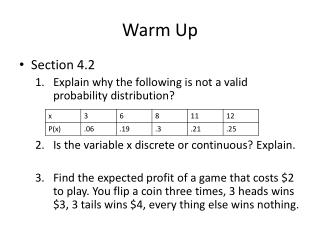Download PresentationWarm Up

Loading in 2 Seconds...

# Warm Up - PowerPoint PPT Presentation

Warm Up. Section 4.2 Explain why the following is not a valid probability distribution? Is the variable x discrete or continuous? Explain. Find the expected profit of a game that costs \$2 to play. You flip a coin three times, 3 heads wins \$3, 3 tails wins \$4, every thing else wins nothing. .I am the owner, or an agent authorized to act on behalf of the owner, of the copyrighted work described.
Download Presentation## Warm Up

An Image/Link below is provided (as is) to download presentation

Download Policy: Content on the Website is provided to you AS IS for your information and personal use and may not be sold / licensed / shared on other websites without getting consent from its author.While downloading, if for some reason you are not able to download a presentation, the publisher may have deleted the file from their server.

- - - - - - - - - - - - - - - - - - - - - - - - - - E N D - - - - - - - - - - - - - - - - - - - - - - - - - -
Presentation Transcript
1. Warm Up • Section 4.2 • Explain why the following is not a valid probability distribution? • Is the variable x discrete or continuous? Explain. • Find the expected profit of a game that costs \$2 to play. You flip a coin three times, 3 heads wins \$3, 3 tails wins \$4, every thing else wins nothing.

2. Warm Up • Section 4.2 • Explain why the following is not a valid probability distribution? Because sum of the probabilities is not 1. • Is the variable x discrete or continuous? Explain. I assume discrete because there are gaps. • Find the expected profit of a game that costs \$2 to play. You flip a coin three times, 3 heads wins \$3, 3 tails wins \$4, every thing else wins nothing.

3. Section 4.4 – Mean, Variance and Standard Deviation for the Binomial Distribution • Remember these values are what will probablyhappen, not what has happened. • Mean:  = n·p • Variance: 2 = n·p·q • Standard Deviation:  = 2 = n·p·q • These values can be used to determine usual values. • Minimum usual value =  - 2 • Maximum usual value =  + 2

4. Example • A report states 40% of cell phone users have Verizon as their carrier. If 50 cell phone users are selected, find the mean, variance and standard deviation, assuming it’s binomial. • Would it unusual to find that 28 of the 50 people use Verizon? Show work.

5. Example • A report states 40% of cell phone users have Verizon as their carrier. If 50 cell phone users are selected, find the mean, variance and standard deviation. •  = .4*50 = 20 2 = .4*50*.6 = 12 •  = √12 = 3.464  3.4 • Would it unusual to find that 28 of the 50 people use Verizon? Show work. • Max usual value = 20 + 2(3.464) = 26.928 • Yes it is unusual since it falls above the maximum unusual value of 26.928